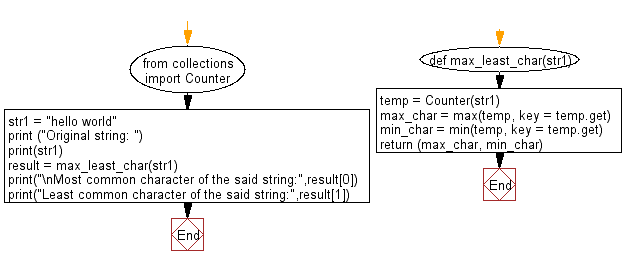﻿ Python: Count most and least common characters in a given string - w3resource

# Python: Count most and least common characters in a given string

## Python Collections: Exercise-21 with Solution

Write a Python program to count the most and least common characters in a given string.

Sample Solution:

Python Code:

``````from collections import Counter
def max_least_char(str1):
temp = Counter(str1)
max_char = max(temp, key = temp.get)
min_char = min(temp, key = temp.get)
return (max_char, min_char)

str1 = "hello world"
print ("Original string: ")
print(str1)
result = max_least_char(str1)
print("\nMost common character of the said string:",result)
print("Least common character of the said string:",result)
```
```

Sample Output:

```Original string:
hello world

Most common character of the said string: l
Least common character of the said string: h
```

Flowchart:## Visualize Python code execution:

The following tool visualize what the computer is doing step-by-step as it executes the said program:

Python Code Editor:

Have another way to solve this solution? Contribute your code (and comments) through Disqus.

What is the difficulty level of this exercise?

Test your Programming skills with w3resource's quiz.

﻿

## Python: Tips of the Day

Maps the values of a list to a dictionary using a function, where the key-value pairs consist of the original value as the key and the result of the function as the value:

Example:

```def tips_map_dictionary(itr, fn):
ret = {}
for a in itr:
ret[a] = fn(a)
return ret
print(tips_map_dictionary([2,4,6], lambda a: a * a))
```

Output:

```{2: 4, 4: 16, 6: 36}
```

We are closing our Disqus commenting system for some maintenanace issues. You may write to us at reach[at]yahoo[dot]com or visit us at Facebook